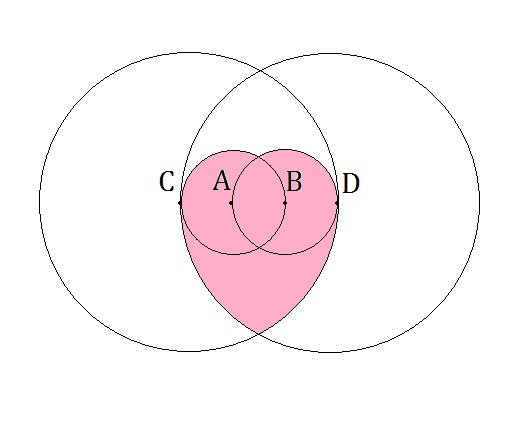# Love Circles

Geometry Level 4The points $A$, $B$, $C$, $D$ are the centers of the circles as shown above. The circles $A$ and $B$ each have a radius of length 1 cm. and pass through each other's center. Similarly, the circles $C$ and $D$ each have a radius of length 3 cm. and also pass through each other's center, inscribing the smaller circles inside the overlapping portion.

What is the area of the pink section (in $\text{cm}^{2}$)? Give your answer in the nearest 3 decimal places.

×